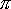QUESTION #432

# Is there a relationship between electrical conductivity and thermal conductivity?

Asked by: Darell Hayes

There is a relationship for metals and it is known as the Wiedemann-Franz law. Metals are good electrical conductors because there are lots of free charges in them. The free charges are usually negative electrons, but in some metals, e.g., tungsten, they are positive 'holes.' For purposes of discussion, let's assume we have free electron charges.

When a voltage difference exists between two points in a metal, it creates an electric field which causes the electrons to move, i.e., it causes a current. Of course, the electrons bump into some of the stationary atoms (actually, 'ion cores') of the metal and this frictional 'resistance' tends to slow them down. The resistance depends on the specific type of metal we're dealing with. E.g., the friction in silver is much less than it is in iron. The greater the distance an electron can travel without bumping into an ion core, the smaller is the resistance, i.e., the greater is the electrical conductivity. The average distance an electron can travel without colliding is called the 'mean free path.' But there's another factor at work too. The electrons which are free to respond to the electric field have a thermal speed a sizable percentage of the speed of light, but since they travel randomly with this high speed, they go nowhere on average, i.e., this thermal speed itself doesn't create any current.

The thermal conductivity of this metal is, like electrical conductivity, determined largely by the free electrons. Suppose now that the metal has different temperatures at its ends. The electrons are moving slightly faster at the hot end and slower at the cool end. The faster electrons transmit energy to the cooler, slower ones by colliding with them, and just as for electrical conductivity, the longer the mean free path, the faster the energy can be transmitted, i.e., the greater the thermal conductivity. But the rate is also determined by the very high thermal speed'the higher the speed, the more rapidly does heat energy flow(i.e., the more rapidly collisions occur). In fact, the thermal conductivity is directly proportional to the product of the mean free path and thermal speed.

Both thermal and electrical conductivity depend in the same way on not just the mean free path, but also on other properties such as electron mass and even the number of free electrons per unit volume. But as we have seen, they depend differently on the thermal speed of the electrons'electrical conductivity is inversely proportional to it and thermal conductivity is directly proportional to it. The upshot is that the ratio of thermal to electrical conductivity depends primarily on the square of the thermal speed. But this square is proportional to the temperature, with the result that the ratio depends on temperature, T, and two physical constants: Boltzmann's constant, k, and the electron charge, e. Boltzmann's constant is, in this context, a measure of how much kinetic energy an electron has per degree of temperature.

Putting it all together, the ratio of thermal to electrical conductivity is:

(2 / 3 ) * ( (k/e)2 ) * T

the value of the constant multiplying T being: 2.45x10-8 W-ohm-K-squared.
Answered by: Frank Munley, Ph.D., Associate Professor, Physics, Roanoke College